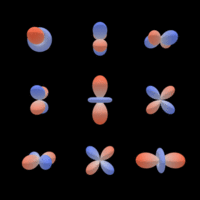# e3nn / e3nn

A modular framework for neural networks with Euclidean symmetry
Python Cuda C++

## Latest commit

```* add LearnableBispectrum

* remove lin to use learnabletensorproduct

* add input to final tensorproduct or dot product

* simplify dot product as LearnableTensorProduct```
Latest commit 665d1e4 Apr 3, 2020

## Files

Type Name Latest commit message Commit time
Failed to load latest commit information..github/workflows Apr 2, 2020e3nn Apr 3, 2020examples Apr 1, 2020src/real_spherical_harmonics Nov 14, 2019tests Apr 2, 2020.gitignore Jul 17, 2019LICENSEREADME.md Mar 23, 2020requirements.txt Feb 26, 2020setup.py Feb 19, 2020

# E3NN

The group E(3) is the group of 3 dimensional rotations, translations and mirror. This library aims to create E(3) equivariant convolutional neural networks.## Example

```from functools import partial
import torch
from e3nn.kernel import Kernel
from e3nn.point.operations import Convolution
from e3nn.util.plot import plot_sh_signal
import matplotlib.pyplot as plt

# Radial model:  R -> R^d
# Projection on cos^2 basis functions followed by a fully connected network

# kernel: composed on a radial part that contains the learned parameters
#  and an angular part given by the spherical hamonics and the Clebsch-Gordan coefficients

# Define input and output representations
Rs_in = [(1, 0)]  # one scalar
Rs_out = [(1, l) for l in range(10)]

# Use the kernel to define a convolution operation
conv = Convolution(K, Rs_in, Rs_out)

n = 3  # number of points
features = torch.ones(1, n, 1)
geometry = torch.randn(1, n, 3)

features = conv(features, geometry)```

## Hierarchy

• `e3nn` contains the library
• `e3nn/o3.py` defines all the needed mathematical functions
• `e3nn/image` contains voxels linear operations
• `e3nn/point` contains points linear operations
• `e3nn/non_linearities` non linearities operations
• `examples` simple scripts and experiments

## Installation

1. install pytorch
2. `pip install git+https://github.com/AMLab-Amsterdam/lie_learn`
3. `pip install git+https://github.com/e3nn/e3nn`

## Citing``````@software{e3nn_2020_3723557,
author       = {Mario Geiger and
Tess Smidt and
Benjamin K. Miller and
Wouter Boomsma and
Kostiantyn Lapchevskyi and
Maurice Weiler and
Michał Tyszkiewicz and
Jes Frellsen},
title        = {github.com/e3nn/e3nn},
month        = mar,
year         = 2020,
publisher    = {Zenodo},
version      = {v0.3-alpha},
doi          = {10.5281/zenodo.3723557},
url          = {https://doi.org/10.5281/zenodo.3723557}
}
``````
You can’t perform that action at this time.